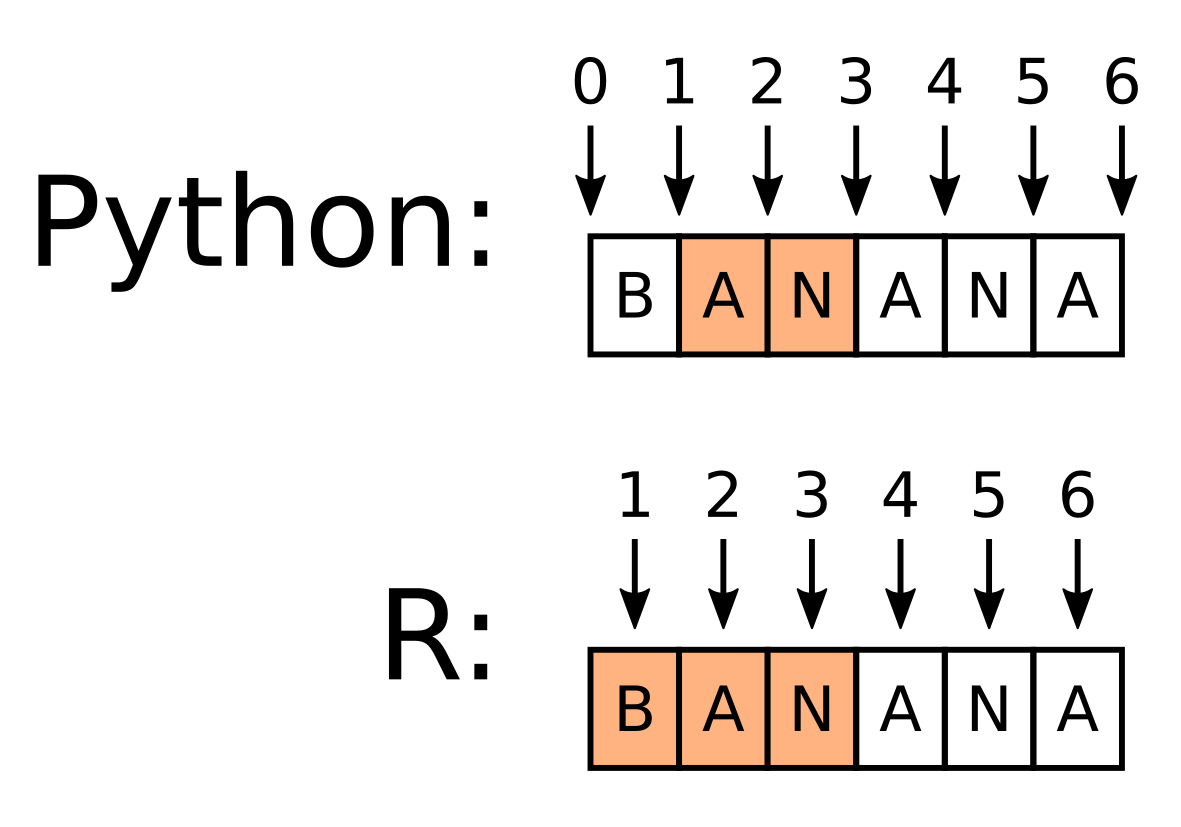To access elements in an array or matrix, programming languages typically implement one of two addressing schemes. One of the schemes uses one-based numbering with closed intervals, the other scheme uses zero-based numbering with half-open (also known as half-closed) intervals.

R, the popular programming language for statistical analysis, uses one-based numbering with closed intervals. One-based means that the first element of the array has the index 1. Closed means that the start and end limits of the interval are both included. The result is that a “slice” [1:3] of any R array will include the first, second and third elements. The first element is included because it has the index 1, and the third element is included because the interval is closed.

Python, a language popular for scientific computing and general programming, uses zero-based numbering with half-open intervals. Zero-based means that the first element of the array has the index 0. Half-open means that only the start limit of the interval is included, and the end limit is excluded. The result is that a slice [1:3] of any Python array will include the second and third elements. The second element is included because it has the index 1, and the fourth element is excluded because the interval is half-open.

You can visualize the different schemes as follows; the indices in R point directly to each array element, whereas the indices in Python point to the gaps between each array element. In both cases then you can visualize the intervals as including all elements between the pointers:While the addressing scheme used by R is more intuitive, the scheme used by Python makes more sense for a programming language. Consider chopping an array in two, in this case into “BAN” and “ANA”. In Python the array can be sliced in two at the index 3: [0:3] and [3:6]. However in R, the slices must be specified to end and begin at different indicies: [1:3] and [3 + 1:6]. Off-by-one errors are extremely common in programming, but they are easier to avoid with the addressing scheme used by Python (and C, and many other languages).